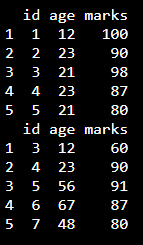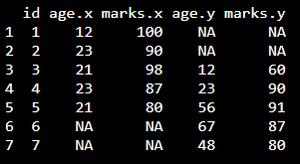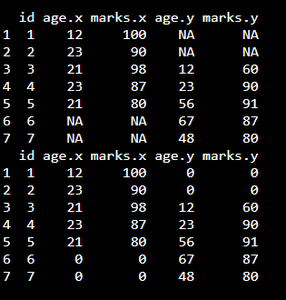Open in App
Not now

# Merge Two Unequal DataFrames and Replace NA with 0 in R

• Last Updated : 29 Sep, 2021

In this article we are going to merge two unequal dataframes and then replace NA values in the resultant dataframe with 0 using R programming language.

Dataframes in use:## Merging the dataframes

We can merge the two dataframes by using merge() function.

Syntax:

merge(dataframe1, dataframe2,   by = “column”,all = TRUE)

where,

• dataframe1 is the first dataframe
• dataframe2 is the second dataframe
• column is the column name to be merged based on this column

Example: R program to merge the dataframes by id column

## R

 `# create dataframe1 with 3 columns``data1 = ``data.frame``(id=``c``(1, 2, 3, 4, 5),``                   ``age=``c``(12, 23, 21, 23, 21),``                   ``marks=``c``(100, 90, 98, 87, 80))` `# create dataframe2 with 3 columns``data2 = ``data.frame``(id=``c``(3, 4, 5, 6, 7),``                   ``age=``c``(12, 23, 56, 67, 48),``                   ``marks=``c``(60, 90, 91, 87, 80))` `# merge the dataframes by id column``data = ``merge``(data1, data2, by=``'id'``, all=``TRUE``)` `# display merged dataframe``print``(data)`

Output:## Replacing NA with 0 in dataframe

We can replace NA by using is.na() function. By setting data to 0 inside the index the job can be easily done.

Syntax:

data[is.na(data)] = 0

Where, data is the merged dataframe with NA values

Example: R program to replace NA with 0

## R

 `# create dataframe1 with 3 columns``data1 = ``data.frame``(id=``c``(1, 2, 3, 4, 5),``                   ``age=``c``(12, 23, 21, 23, 21),``                   ``marks=``c``(100, 90, 98, 87, 80))` `# create dataframe2 with 3 columns``data2 = ``data.frame``(id=``c``(3, 4, 5, 6, 7),``                   ``age=``c``(12, 23, 56, 67, 48),``                   ``marks=``c``(60, 90, 91, 87, 80))` `# merge the dataframes by id column``data = ``merge``(data1, data2, by=``'id'``, all=``TRUE``)` `# display merged dataframe``print``(data)` `# Replace NA with 0``data[``is.na``(data)] = 0` `print``(data)`

Output:My Personal Notes arrow_drop_up